如何使用MATLAB绘制实时温度图表

二维码 36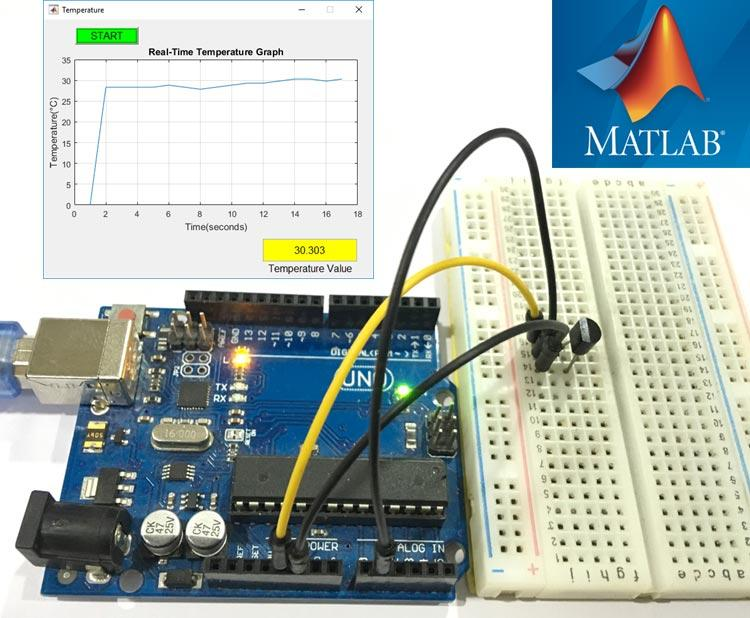MATLAB入门：快速入门将

Arduino与MATLAB连接 -闪烁LED

 guide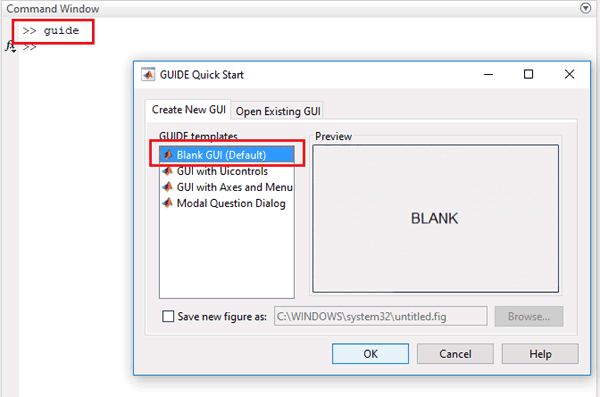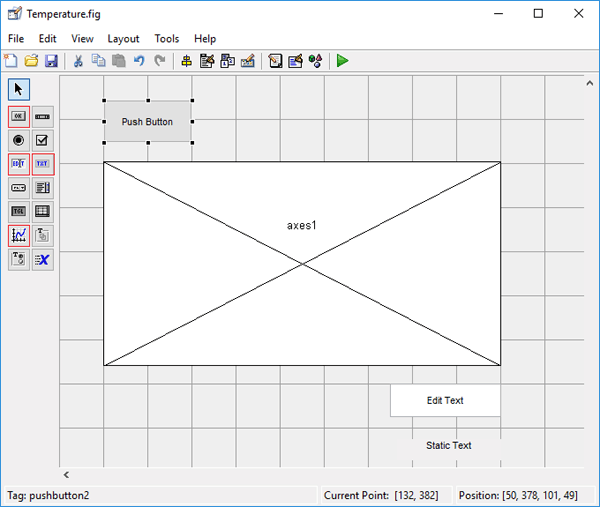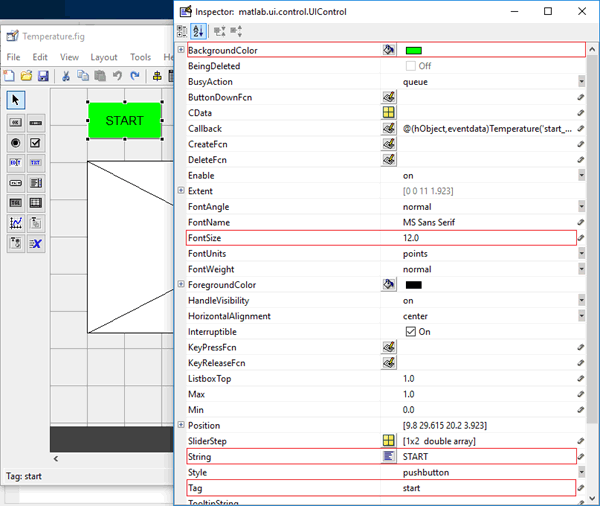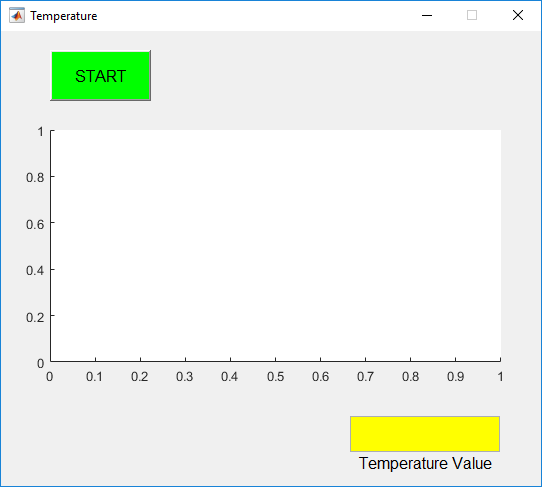clear all;global a;a = arduino();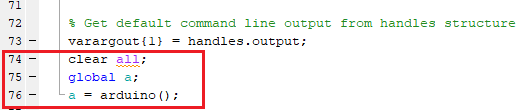x = 0;go = true;global a;while go value = readVoltage(a,'A1');temp = (value*100);disp(temp);x = [x temp];plot(handles.axes1,x);grid on;xlabel('Time(seconds)')ylabel('Temperature(°C)');title('Real-Time Temperature Graph');drawnowset(handles.edit1,'String',num2str(temp));pause(1);end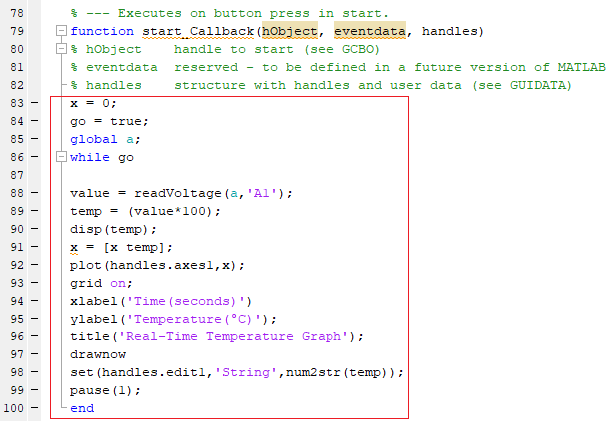x = 0;go = true;global a;

 temp = (value*100);

"网格上" 用于在图形的 "网格" 视图上, "xlabel"、"ylabel" 和 "title" 用于命名 x 轴、y 轴和标题。

 plot(handles.axes1,x);grid on;xlabel('Time(seconds)')ylabel('Temperature(°C)');title('Real-Time Temperature Graph');

'Drawnow' 用于实时更新图形表示。

 drawnow

 set(handles.edit1,'String',num2str(temp));

MATLAB安装笔记本电脑（首选项：R2016a或以上版本）

Arduino UNO

LM35  - 温度传感器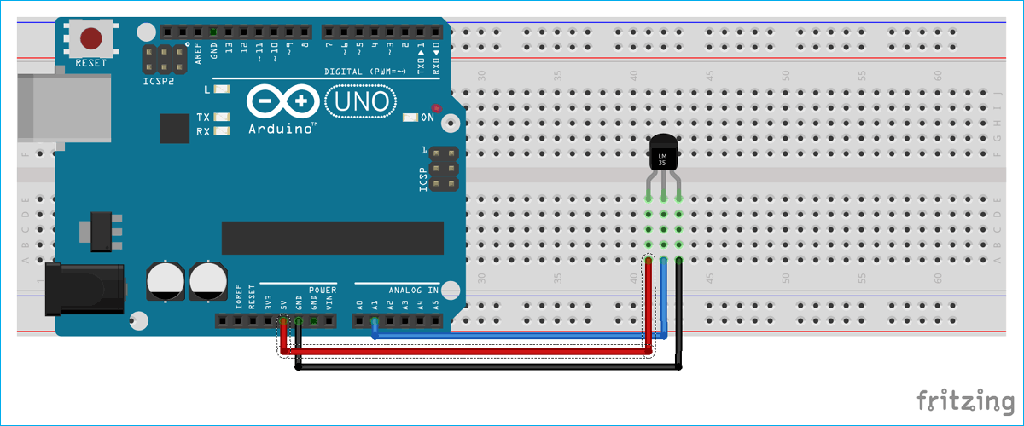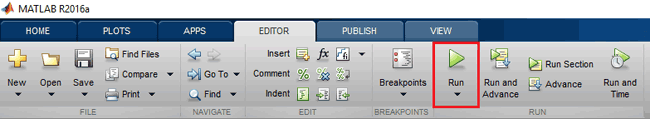MATLAB可能需要几秒钟的时间来响应，不要点击任何GUI按钮，直到MATLAB在左下角显示忙消息，如下所示，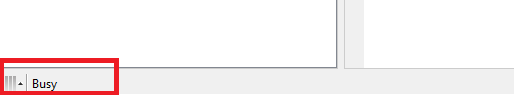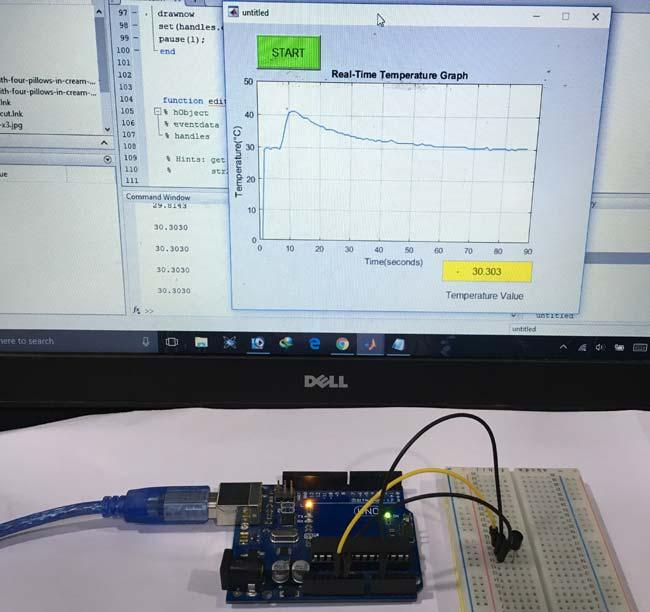MATLAB Code:function varargout = Temperature(varargin)gui_Singleton = 1;gui_State = struct('gui_Name',       mfilename, ...                              'gui_Singleton',  gui_Singleton, ...                              'gui_OpeningFcn', @Temperature_OpeningFcn, ...                              'gui_OutputFcn',  @Temperature_OutputFcn, ...                              'gui_LayoutFcn',  [] , ...                              'gui_Callback',   []);if nargin && ischar(varargin{1})              gui_State.gui_Callback = str2func(varargin{1});endif nargout           [varargout{1:nargout}] = gui_mainfcn(gui_State, varargin{:});else             gui_mainfcn(gui_State, varargin{:});endfunction Temperature_OpeningFcn(hObject, eventdata, handles, varargin)handles.output = hObject;guidata(hObject, handles);function varargout = Temperature_OutputFcn(hObject, eventdata, handles) varargout{1} = handles.output;clear all;global a;a = arduino;function start_Callback(hObject, eventdata, handles)x = 0;go = true;global a;while go                  value = readVoltage(a,'A1');temp = (value*100);disp(temp);x = [x temp];plot(handles.axes1,x);grid on;xlabel('Time(seconds)')ylabel('Temperature(°C)');title('Real-Time Temperature Graph');drawnowset(handles.edit1,'String',num2str(temp));pause(1);end function edit1_Callback(hObject, eventdata, handles)function edit1_CreateFcn(hObject, eventdata, handles)if ispc && isequal(get(hObject,'BackgroundColor'),   get(0,'defaultUicontrolBackgroundColor'))   set(hObject,'BackgroundColor','white');end